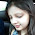Dear students,Numerical Ability or Quantitative Aptitude Section has given heebie-jeebies to the aspirants when they appear for a banking examination. As the level of every other section is only getting complex and convoluted, there is no doubt that this section, too, makes your blood run cold. The questions asked in this section are calculative and very time-consuming. But once dealt with proper strategy, speed, and accuracy, this section can get you the maximum marks in the examination. Following is the Quantitative Aptitude quiz to help you practice with the best of latest pattern questions.

Directions (1-10): In each of the following questions two equations are given. You have to solve the equations and
Give answer –
(a) if x < y
(b) if x ≤ y
(c) relationship between x and y cannot be determined
(d) if x ≥ y
(e) if x > y

Q1. I. 8x² + 18x + 9 = 0
II. 4y² + 19y + 21 = 0

Q2. I. 3x² + 16x + 21 = 0
II. 6y² + 17y + 12 = 0

Q3. I. 16x² + 20x + 6 = 0
II. 10y² + 38y + 24 = 0

Q4. I. 8x² + 6x = 5
II.12y² – 22y + 8 = 0

Q5. I. 17x² + 48x = 9
II. 13y² = 32y – 12

Solutions (1-5):

Q6. I. 8x² + 26x + 15 = 0
II. 4y² + 24y + 35 = 0

Q7. I. 2x² + 9x + 9 = 0
II. 2y² + 17y + 36 = 0

Q8. I. 5x² + 29x + 20 = 0
II. 25y² + 25y + 6 = 0

Q9. I. 3x² – 16x + 21 = 0
II. 3y² – 28y + 65 = 0

Solutions (6-10):

Directions (11-15): In the following questions two equations numbered I and II are given. You have to solve both the equations and–

Solutions (11-15):#### 4 comments:

1.last question is wrong

2.last question ans cannot be establised

3.4.Last Question is P=Q